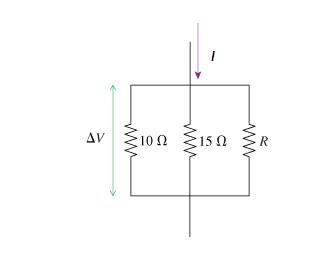# What is the value of resistor R in the figure(Figure 1) if V=7V and I=6A?

edited

What is the value of resistor R in the figure(Figure 1) if V=7V and I=6A?

What is the value of resistor R in the figure(Figure 1) if V=7V and I=6A?

Express your answer using two significant figures.by
selected by

1/10 + 1/15 + 1/R = 1/R(net)

according to Ohm's Law: I*R(net) = V

=> R(net) + V/I => 7/6

=> 1/R(net) = 6/7 = 1/10 + 1/15 + 1/R

=> 6/7 - 1/10 - 1/15 = 1/R

=> 0.69047619047619047619047619047619 (used Windows Calculator)

=> R = 0.69047619047619047619047619047619 ^ -1

=> R = 1.4482758620

=> R = 1.44 ohms

Lorem ipsum dolor sit amet, consectetur adipiscing elit, sed do eiusmod tempor incididunt ut labore et dolore magna aliqua. Ut enim ad minim veniam, quis nostrud exercitation ullamco laboris nisi ut aliquip ex ea commodo consequat. Duis aute irure dolor in reprehenderit in voluptate velit esse cillum dolore eu fugiat.
by

V = 7 V

I = 6 A

Req = V / I = 7/6 Ω

Req = Equivalent resistance of the combination

of 10 Ω, 15 Ω and R Ω connected in parallel

=> 1/Req = (1/10 + 1/15 )+ 1/R

=> 1/(7/6) = 1/6 + 1/R

=> 6/7 = 1/6 + 1/R

=> 1/R = 6/7 - 1/6 = 29/42

=> R = 42/29 = 1.4 Ω

Lorem ipsum dolor sit amet, consectetur adipiscing elit, sed do eiusmod tempor incididunt ut labore et dolore magna aliqua. Ut enim ad minim veniam, quis nostrud exercitation ullamco laboris nisi ut aliquip ex ea commodo consequat. Duis aute irure dolor in reprehenderit in voluptate velit esse cillum dolore eu fugiat.
by

v=IR

7=6*R

R=7/6

R=1.17ohm

Lorem ipsum dolor sit amet, consectetur adipiscing elit, sed do eiusmod tempor incididunt ut labore et dolore magna aliqua. Ut enim ad minim veniam, quis nostrud exercitation ullamco laboris nisi ut aliquip ex ea commodo consequat. Duis aute irure dolor in reprehenderit in voluptate velit esse cillum dolore eu fugiat.

+1 vote
+1 vote